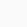# How to use close interval [x] in LaTeX?

SymbolClose interval
Type of symbolMathematics
Package (requirement)No
ArgumentYes
Latex command\left[arg1,arg2\right]
Example\left[arg1,arg2\right] → [arg1,arg2]

You can use the bracket key [, ] on the keyboard to write close intervals in LaTeX. But, in my opinion, this is not the best method when you are using fractions. The bracket size does not adjust automatically if you use the bracket key on the keyboard, below are some examples.

\documentclass{article}
\begin{document}
$[x_1,\;x_n]$
$[a,\;b] = \{x\mid a\le x\le b\}$
$[\frac{1}{y_1},\;\frac{1}{y_2}]$
\end{document}

Output :If you use fractions, the best method is to use the \left[arg1,arg2\right] command, which will automatically adjust the size of your bracket based on the size of the fraction.

\documentclass{article}
\begin{document}
$\left[\frac{1}{y_2},\;\frac{1}{y_1}\right]$
$\left[\frac{1}{a_2},\frac{1}{a_1}\right]$
\end{document}

Output :Scroll to Top